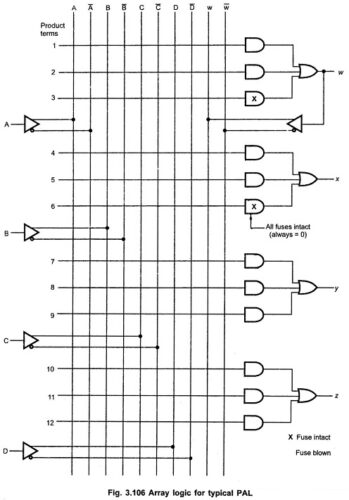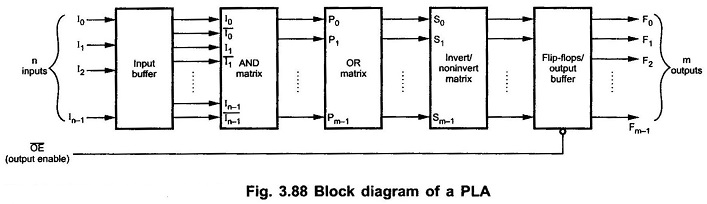# Digital Integrated Circuits

## Synchronous Sequential Circuits

Synchronous Sequential Circuits or Clocked Sequential Circuits: In Synchronous Sequential Circuits or clocked sequential circuits, clocked flip-flops are used as memory elements, which change their individual states in synchronism with the periodic clock signal. Therefore, the change in states of flip-flops and change in state of the entire circuit occurs at the transition of the …

## Performance of CMOS Gates

Performance of CMOS Gates: The Performance of CMOS Gates which based on following different categories namely, Operating Speed : Slower than TTL series. Approximately 25 to 100 ns depending on the subfamily of CMOS Inverter Circuit. It also depends on the power supply voltage. Voltage Levels and Noise Margins : The voltage levels for CMOS …

## CMOS NOR Gate Circuit

CMOS NOR Gate Circuit: Fig. 3.4 shows 2-input CMOS NOR Gate Circuit. Here, P-channel MOSFETs Q1 and Q2 are connected in series and N-channel MOSFETs Q3 and Q4 are connected in parallel. Like NAND circuit, this circuit can be analyzed by realizing that a LOW at any input turns ON its corresponding P-channel MOSFET and turns OFF …

## CMOS NAND Gate Circuit Diagram

CMOS NAND Gate Circuit Diagram: Fig. 3.3 shows CMOS NAND Gate Circuit Diagram 2-input NAND gate. It consists of two P-channel MOSFETs, Q1 and Q2, connected in parallel and two N-channel MOSFETs, Q3 and Q4 connected in series. P-channel MOSFET is ON when its gate voltage is negative with respect to its source whereas N-channel …

Parallel Adder and Subtractor: Parallel Adder and Subtractor which consists of three categories, namely,    n Bit Parallel Adder,Binary Parallel Adder and n Bit Parallel Subtractor. n Bit Parallel Adder: We have seen, a single full-adder is capable of adding two one-bit numbers and an input carry. In order to add binary numbers with more …

## Half Subtractor and Full Subtractor Circuit

Half Subtractor and Full Subtractor Circuit: Subtractor are divided into two categories namely, half subtractor and full subtractor circuit. Half Subtractor Circuit: The Half Subtractor Circuit consists of four possible elementary operations, namely, In all operations, each subtrahend bit is subtracted from the minuend bit. In case of second operation the minuend bit is smaller …

Half Adder and Full Adder Circuit: Adders are divided into two categories namely, half adder and full adder circuit. Half Adder Circuit: The Half Adder Circuit operation needs two binary inputs : augend and addend bits; and two binary outputs : sum and carry. The half adder truth table shown in 3.6 gives the relation …

## Programmable Array Logic (PAL)Programmable Array Logic (PAL): We have seen that PLA device with a Programmable AND array and Programmable OR array. However, Programmable Array Logic (PAL) is a programmable logic device with a fixed OR array and a programmable AND array. Because only AND gates are programmable , the PAL is easier to program, but is not …

## Programmable Logic Array (PLA)Programmable Logic Array (PLA) | Block Diagram of PLA: The combinational circuit do not use all the minterms every time. Occasionally, they have don’t care conditions. Don’t care condition when implemented with a ROM becomes an address input that will never occur. The result is that not all the bit patterns available in the ROM …

Scroll to Top